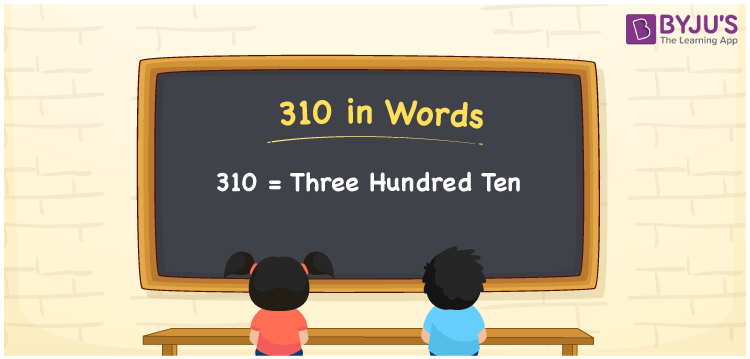# 310 in Words

310 in words is written as Three Hundred Ten. Using a place value chart we can convert the number 310 into words. If you have Rs. 310, then you can say, “I have Rupees Three Hundred Ten with me”. 310 is a cardinal number since it represents an exact quantity. Let us learn how to spell the number 310 in English words in this article.

 310 in Words Three Hundred Ten Three Hundred Ten in numerical form 310

## 310 in English Words

Generally, we write numbers in words with the help of the English alphabet. Hence, we can write the number 310 in words as Three Hundred Ten.## How to Write 310 in Words?

Place value chart helps students to understand and express the numbers in a better way. Since 310 is a three-digit number. So, let us make a chart of 3 columns as represented in the below table.

 Hundreds Tens Ones 3 1 0

Therefore, we can write the expanded form as:

3 x Hundred + 1 x Ten + 0 x One

= 3 x 100 + 1 x 10 + 0 x 1

= 300 + 10 + 0

= 310

= Three Hundred Ten

Hence, 310 in words is written as Three Hundred Ten

Interesting way of writing 310 in words

3 = Three

31 = Thirty-One

310 = Three Hundred Ten

Thus, the word form of the number 310 is Three Hundred Ten

310 is a natural number that is the successor of 309 and the predecessor of 311

• 310 in words – Three Hundred Ten
• Is 310 an odd number? – No
• Is 310 an even number? – Yes
• Is 310 a perfect square number? – No
• Is 310 a perfect cube number? – No
• Is 310 a prime number? – No
• Is 310 a composite number? – Yes

## Frequently Asked Questions on 310 in Words

### How to write 310 in words?

310 in words is written as Three Hundred Ten.

### Simplify 200 + 110, and express in words.

Simplifying 200 + 110, we get 310. Hence, 310 in words is Three Hundred Ten.

### Is 310 a composite number?

Yes, 310 is a composite number.